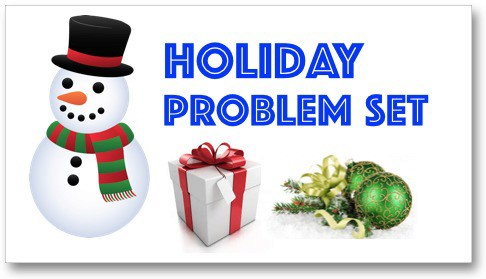# Holiday Problem Set

By | Added Dec 23, 2014Want to test your math skills and get into the holiday spirit? Try our Christmas and Hannukah word problems!

## Lower Elementary:Question: Charlene, Ryan, and Jayleen are hanging ornaments on the Christmas tree. Charlene hung 12 ornaments on the tree. Ryan hung 11 ornaments on the tree. Jayleen hung 15 ornaments on the tree. How many ornaments did they hang on the tree altogether?

Solution: To find the total number of ornaments that they hung on the tree, we add the number of ornaments that each of them put on the tree. 12 + 11 + 15. Notice that 12 + 11 can be done by doing doubles minus 1. 12 doubled is 24, so 12 + 11 = 23. So, 12 + 11 + 15 = 23 + 15 = 38. They hung 38 ornaments on the tree.

## Upper Elementary:Question: For Hanukkah, Larry needs to buy candles. The candles need to stay lit for at least 1 hour. Every inch of a candle can last 12 minutes. How long do the candles need to be in order to burn for an hour?

Solution: We can solve this problem by counting in groups. We know that one inch of the candle can last 12 minutes. That means that 2 inches can last 24 minutes, 3 inches can last 36 minutes, 4 inches can last 48 minutes, and 5 inches can last 60 minutes or 1 hour. So, that means the candles need to be 5 inches to burn for 1 hour.

##Question: Walter is wrapping a gift box. He needed 486 square inches of wrapping paper to cover the whole box. If the box is a cube, what is the volume of the box?

Solution: The shape of the gift box is a cube. The surface area of a cube is the sum of the area of each face. Since a cube has six faces, each face has an area of 81 square inches. Since each face is a square the side length is 9 inches. The volume of a cube is the side length raised to the third power. 93 = 9 × 9 × 9 = 81 × 9 = 729. The volume of the cube is 729 cubic inches. You can also find the side length and volume by using the formulas. The surface area of a cube is 6L2, where L is the length.
486 = 6L2
Divide by 6.
81 = L2
9 = L
The side length of the cube is 9 inches. The volume of a cube is V = L3 = 93 = 9 × 9 × 9 = 81 × 9 = 729. The volume of the cube is 729 cubic inches.

## Algebra and Up:Question: A group of 7 friends decide to do gift exchange where each of them bring a present and then they pull a number from a hat to determine the order in which they will pick the gift. How many different orders are there for the friends to pick gifts?